﻿ A Fixed Point Result of Expanding Mappings in Complete Cone Metric Spaces

K. Prudhvi

## A Fixed Point Result of Expanding Mappings in Complete Cone Metric Spaces

Department of Mathematics, University College of Science, Saifabad, Osmania University, Hyderabad, Telangana State, India

### Abstract

In this paper, we prove a fixed point theorem for expanding onto self-mappings in complete cone metric spaces. Our results improve and extend some comparable results in the literature.

• K. Prudhvi. A Fixed Point Result of Expanding Mappings in Complete Cone Metric Spaces. Journal of Mathematical Sciences and Applications. Vol. 3, No. 1, 2015, pp 1-2. http://pubs.sciepub.com/jmsa/3/1/1
• Prudhvi, K.. "A Fixed Point Result of Expanding Mappings in Complete Cone Metric Spaces." Journal of Mathematical Sciences and Applications 3.1 (2015): 1-2.
• Prudhvi, K. (2015). A Fixed Point Result of Expanding Mappings in Complete Cone Metric Spaces. Journal of Mathematical Sciences and Applications, 3(1), 1-2.
• Prudhvi, K.. "A Fixed Point Result of Expanding Mappings in Complete Cone Metric Spaces." Journal of Mathematical Sciences and Applications 3, no. 1 (2015): 1-2.

 Import into BibTeX Import into EndNote Import into RefMan Import into RefWorks

### 1. Introduction

In 2007, Huang and Zhang  introduced cone metric spaces replacing the real numbers by an ordered Banach space, and they have proved some fixed point theorems for self-mapping satisfying different types of contractive conditions in cone metric spaces. Later on, many authors have generalized and extended Huang and Zhang  fixed point theorems (see, e.g., [1, 2, 3, 7, 8]). In 1984, the concept of expanding mappings was introduced by Wang et. al. . In 1992, Daffer and Kaneko  defined expanding mappings for pair of mappings in complete metric spaces and proved some fixed point theorems. In 2012, X. Huang, Ch. Zhu and Xi Wen  proved some fixed point theorems for expanding mappings cone metric spaces and they have also extended the results of Daffer and Kaneko . The main aim of this paper is we proved a fixed point theorem for expanding mappings in cone metric spaces, our result extends and improves the results of .

The following definitions and properties are due to Huang and Zhang .

Definition 1.1. Let B be a real Banach space and θ is the zero element of B, P a subset of B. The set P is called a cone if and only if:

(i) P is closed, non–empty and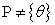;

(ii)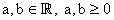,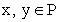implies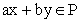;

(iii)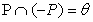.

For a cone P in a Banach space B, define partial orderingwith respect to P byif and only if. We shall writeto indicate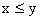but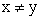, whilewill stand for, where Int P denotes the interior of the set P. This cone P is called an order cone.

Let B be a Banach space and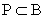be an order cone .The order cone P is called normal if there exists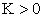such that for all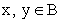,The least positive number K satisfying the above inequality is called the normal constant of P.

Definition 1.2. Let X be a nonempty set of B. Suppose that the map d: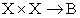satisfies:

(d1)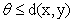for allandif and only if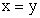;

(d2)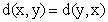for all;(d3)for all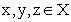.

Then d is called a cone metric on X and (X, d) is called a cone metric space.

Definition 1.3. Let (X, d) be a cone metric space .We say that {xn} is

(i) a Cauchy sequence if for every c in B with, there is N such that for all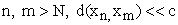;

(ii) a convergent sequence if for any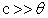, there is an N such that for all, for some fixed x in X. We write(as).

The space (X, d) is called a complete cone metric space if every Cauchy sequence is convergent .

Definition 1.4.  Let (X, d) be a cone metric space and T: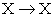, then T is called a expanding mapping, if for every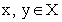there exists a number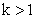such that.

### 2. Main Result

In this section, we prove a fixed point theorem for expanding mappings in complete cone metric spaces.

We prove a Lemma which is useful in the main theorem.

Lemma 2.1. Let (X, d) be a cone metric space and {xn} be a sequence in X. If there exists a number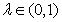such that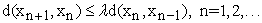(1)

then {xn} is a Cauchy sequence in X.

Proof. By the induction and the condition (1), we have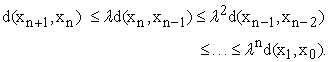For n > mLet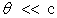be given. Choose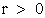such that, where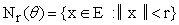. Also choose a natural number N1 such that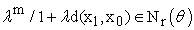, for all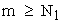. ThusHence, {xn} is a Cauchy sequence in X.

The following theorem improved and extended the Theorem 2.1. of .

Theorem 2.2. Let (X, d) be a complete cone metric space and T:be a surjection. Suppose that there existswithsuch that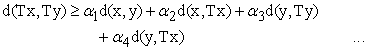(2)

for all. Then T has a fixed point in X.

Proof. By our assumption, it is clear that T is injective. Let F be the inverse mapping of T.

Let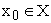, then,We assume thatfor allotherwise, for some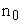, then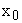is a fixed point of T.

From the condition (2) it follows that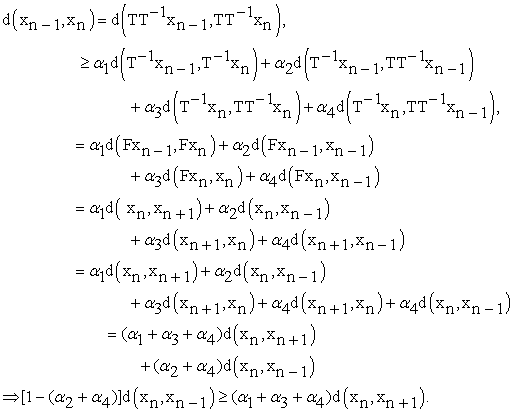If, then

Since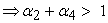.

Then the above inequality implies that a negative number is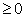, which is not possible.

So,and.

Therefore,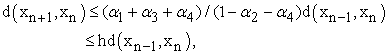where,.

By the Lemma 2.1, we get that {xn} is a Cauchy sequence in X. Since (X, d) is complete, the sequence {xn} converges to a point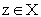. Let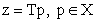, we have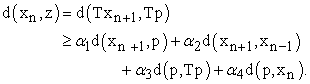Letting, we get that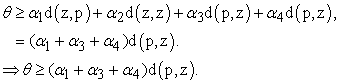That is,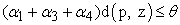.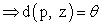. That is,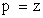.

Therefore,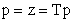.

Therefore, z is a fixed point of T.

Remark 2.3. If we choose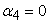in Theorem 2.1, then we get that Theorem 2.1. of .

Remark 2.4. If we chooseandin Theorem 2.1, then we get that Corollary 2.1. of .

### References

  M. Abbas and G. Jungck, Common fixed point results for non commuting mappings without continuity in cone metric spaces, J. Math. Anal. Appl. 341(2008) 416-420.In article View Article  M. Arshad, A. Azam, P. Vetro, Some common fixed point results on cone metric spaces, Fixed Point Theory Appl. (2009)11, Article ID 493965.In article View Article  A. Azam, M. Arshad, I. Beg, Banach contraction principle on cone rectangular metric spaces,Appl. Anal. Discrete Math., 3(2),(2009), 236-241.In article View Article  P. Z. Daffer and H. Kaneko, On expansive mappings, Math. Japon 37 (1992), 733-735.In article  L. G. Huang, X. Zhang, Cone metric spaces and fixed point theorems of contractive mappings, J. Math. Anal. Appl. 332(2) (2007)1468-1476.In article View Article  X. Huang, Ch. Zhu and Xi Wen, Fixed point theorems for expanding mappings in cone metric spaces, Math Reports 14(64),2(2012), 141-148.In article  D. IIic, V. Rakocevic, Common fixed points for maps on cone metric space, J. Math. Anal. Appl. 341(2008) 876-886.In article View Article  Sh. Rezapour and R. Hamlbarani, Some notes on the paper cone metric spaces and fixed point theorem of contractive mappings, J. Math. Anal. Appl. 345(2008), 719-724.In article View Article  S. Z. Wang, B. Y. Li, Z. M. Gao and K. Iseki, Some fixed point theorems for expansion mappings, Math. Japon 29(1984), 631-636.In article